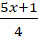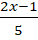### Linear Inequalities 2

Mathematics J.S.S 2 Second Term

Theme: Algebraic Process

Sub Theme: Algebraic Operation

WEEK 8

Linear Inequalities 2

Performance Objectives

Students should be able to;

1. Solving word problems on inequalities
2. Solving word problems involving linear inequalities in one variable

Word Problems on Inequalities

Content

Example 1Solve the given inequality. Present the solution set using interval notation and plot it on a number line.-> 2x - 3
Solution:

We will bring both sides to the common denominator, and then clear all denominators by multiplying by that number. In fact, we can speed up the process by just multiplying both sides by the common denominator. In this case, that common denominator.... View More